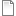# Data-specified association between instances of the same submodel

ModelId:
data_specified_assoc2
SimileVersion:
soc2 Simile version :

This model demonstrates how to set up an association between submodel instances using a data file containing pairs of instances.Category:
Technique
Equations:

Equations in ../Desktop

IDa = [1,2,3]
IDb = [2,3,4]

Equations in Node
ID = index(1)

Equations in Arc
cond1 = (any(ID == [IDa]and ID_0 == [IDb])or any(ID == [IDb]and ID_0 == [IDa]))
where
ID = ../Node/ID (from Node in role1)
ID_0 = ../Node/ID (from Node in role2)
[IDa] = ../IDa

AttachmentSizedata_specified_assoc2.sml28.04 KB
Model tags: